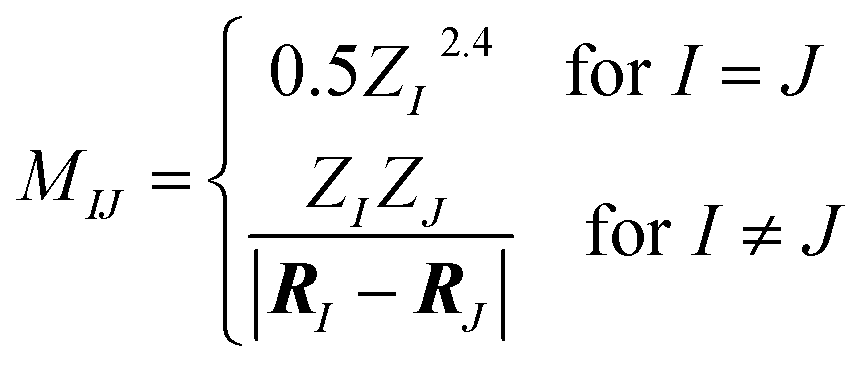You can run this notebook online in a Binder session or view it on Github.

# (Un)Supervised ML Examples¶

The notebook provides two ML examples:

• Unsupervised learning: use manifold learning to understand the structure of the QM7b, QM7b-T, and SN2 Reaction datasets.

• Supervised learning: train a kernel model to predict atomization energies with the ANI-1 dataset, and test it on a COMP6 benchmark.

Begin by connecting to the MolSSI server:

:

import numpy as np
import pandas as pd

import qcportal as ptl

client = ptl.FractalClient()


## Unsupervised learning: manifold learning with QM7b, QM7b-T, and SN2 Reactions¶Manifold learning is a strategy for exposing the structure of high-dimensional datasets through non-linear dimensionality reduction. In this example, we will look at the similarities and differences within and between three ML datasets. Molecules will be fingerprinted by Coulomb matrix features, and manifold learning will be performed with the Uniform Manifold Approximation and Projection (UMAP) method.

:

qm7b = client.get_collection("dataset", "qm7b")
print_info(qm7b)

Name: QM7b

Data Points: 7211
Elements: ['C', 'H', 'Cl', 'N', 'O', 'S']
Labels: ['atomization energy', 'excitation energy', 'lumo', 'ionization potential', 'electron affinity', 'polarizability', 'absorption intensity', 'homo']

Description: Small organic molecules with up to 7 heavy atoms, sampled from GDB-13 and optimized at the PBE0 level of theory. Ground and excited state properties are evaluated at the PBE0, ZINDO, and GW levels of theory. This dataset is also available on quantum-machine.org and qmml.org.
Blum, L. C. & Reymond, J.-L. 970 million druglike small molecules for virtual screening in the chemical universe database GDB-13. JACS, 2009, 131, 8732-8733.
Montavon, G.; Rupp, M.; Gobre, V.; Vazquez-Mayagoitia, A.; Hansen, K.; Tkatchenko, A.; Müller, K.-R. & Von Lilienfeld, O. A. Machine learning of molecular electronic properties in chemical compound space. New J. Phys., 2013, 15, 095003.

### Generating coulomb matrix features¶

Molecule objects contain information about atomic symbols/numbers, geometry, charge, spin, fragments, etc. As an example of using this information, the coulomb_matrix function below computes the (sorted) Coulomb matrix features corresponding to a Molecule. The Coulomb matrix is given by:Coulomb matrix features are not the most sophisticated, but they are simple to implement:

:

import qcelemental
scale = qcelemental.constants.conversion_factor("bohr", "angstrom") * np.sqrt(2)

def coulomb_matrix(mol, size=23):
"""
Compute the sorted Coulomb matrix features corresponding to a molecules

Parameters
----------
mol: Molecule
the molecule whose features are to be computed
size: int, optional
maximum dimension of the Coulomb matrix
"""
natoms = len(mol.atomic_numbers)

# Create the Coulomb matrix
M = np.zeros((natoms, natoms))
for i in range(natoms):
M[i,i] = 0.5 * mol.atomic_numbers[i]**2.4
for j in range(0,i):
M[i,j] = mol.atomic_numbers[i] * mol.atomic_numbers[j] / \
np.linalg.norm(mol.geometry[i,:] - mol.geometry[j,:] * scale )
M[j,i] = M[i,j]

# Sort based on row norm, and zero-pad or truncate to size
order = np.argsort(np.linalg.norm(M, axis=0))[::-1][:size]
ret = np.zeros((size, size))
for i in range(min(natoms, size)):
for j in range(min(natoms, size)):
ret[i,j] = M[order[i], order[j]]

# Flatten upper triangle to 1D
return ret[np.triu_indices(size)]


Compute the Coulomb matrix feature for each molecule. The code below creates a new column in the DataFrame containing the features.

:

qm7b_mols = qm7b.get_molecules()
qm7b_mols["feature"] = [coulomb_matrix(molecule) for molecule in qm7b_mols["molecule"]]
qm7b_mols

:

molecule feature
index
0 Geometry (in Angstrom), charge = 0.0, mult... [36.85810519942594, 3.0602011490558505, 3.0553...
1 Geometry (in Angstrom), charge = 0.0, mult... [36.85810519942594, 10.707116135022035, 3.0751...
10 Geometry (in Angstrom), charge = 0.0, mult... [53.3587073998281, 12.483520879461704, 7.74603...
100 Geometry (in Angstrom), charge = 0.0, mult... [53.3587073998281, 7.416502178204354, 10.61293...
1000 Geometry (in Angstrom), charge = 0.0, mult... [73.51669471981023, 10.524193502952423, 4.7371...
... ... ...
995 Geometry (in Angstrom), charge = 0.0, mult... [73.51669471981023, 12.945983223622989, 6.2342...
996 Geometry (in Angstrom), charge = 0.0, mult... [73.51669471981023, 17.492583403086307, 17.890...
997 Geometry (in Angstrom), charge = 0.0, mult... [73.51669471981023, 13.44823763751797, 8.96043...
998 Geometry (in Angstrom), charge = 0.0, mult... [73.51669471981023, 13.374609104346993, 8.7642...
999 Geometry (in Angstrom), charge = 0.0, mult... [73.51669471981023, 12.325415318305255, 13.542...

7211 rows × 2 columns

### Manifold learning with UMAP¶

Often in ML, features live in a high-dimensional space, but may have low dimensional structure. To examine this, we use UMAP to reduce the Coulomb matrix features of QM7b onto two dimensions:

:

import umap
import warnings; warnings.simplefilter('ignore')  # supress numba warnings

reducer = umap.UMAP()
X = np.vstack(qm7b_mols["feature"])  # format features into matrix for UMAP
embedding = reducer.fit_transform(X)
print(f"Feature matrix shape: {X.shape}")
print(f"UMAP embedding shape: {embedding.shape}")

Feature matrix shape: (7211, 276)
UMAP embedding shape: (7211, 2)

:

import plotly.express as px

qm7b_mols["Embedding Dim. 1"] = embedding[:, 0]
qm7b_mols["Embedding Dim. 2"] = embedding[:, 1]

fig = px.scatter(qm7b_mols, x="Embedding Dim. 1", y="Embedding Dim. 2", title="UMAP Embedding of QM7b")
fig.show()